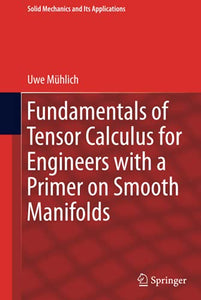# Fundamentals of Tensor Calculus for Engineers with a Primer on Smooth Manifolds

Uwe Mühlich

Format: Print Book

ISBN: 9783319562636

• SGD 202.79
Unit price per
• Save SGD 22.53
Tax included.

Will not ship until

This book presents the fundamentals of modern tensor calculus for students in engineering and applied physics, emphasizing those aspects that are crucial for applying tensor calculus safely in Euclidian space and for grasping the very essence of the smooth manifold concept.After introducing the subject, it provides a brief exposition on point set topology to familiarize readers with the subject, especially with those topics required in later chapters.It then describes the finite dimensional real vector space and its dual, focusing on the usefulness of the latter for encoding duality concepts in physics. Moreover, it introduces tensors as objects that encode linear mappings and discusses affine and Euclidean spaces. Tensor analysis is explored first in Euclidean space, starting from a generalization of the concept of differentiability and proceeding towards concepts such as directional derivative, covariant derivative and integration based on differential forms. The final chapter addresses the role of smooth manifolds in modeling spaces other than Euclidean space, particularly the concepts of smooth atlas and tangent space, which are crucial to understanding the topic. Two of the most important concepts, namely the tangent bundle and the Lie derivative, are subsequently worked out.

Publication Year: 2017
Imprint: Springer International Publishing
Format: H
Weight (Gram): 3376